Tutorial

# Natural Language Processing — An Overview of Key Algorithms and Their EvolutionI. Rules and progression to machine learning including probabilistic modeling (Naïve Bayes and Conditional Random Fields)

The field of Natural Language processing has come a long way thanks to the contributions of many people in its advancement. To fully appreciate this evolution, it’s important to understand some of the key algorithms and factors driving their development. In this post we’ll provide some context into this evolution by providing an overview and progression of some of the core NLP techniques with their pros and cons, helping us to understand their progression. The post is in 2 sections: Section I talks about the Rules and probabilistic based NLP modeling; Section II will talk about the recent advances. Most of the work on NLP in the beginning (pre-1980s) were rule-based. Rule based systems like Regular expressions (RE) are based on pattern matching on text and work well in certain situations. For e.g. if we must find all the punctuations in a sentence, RE can be very useful: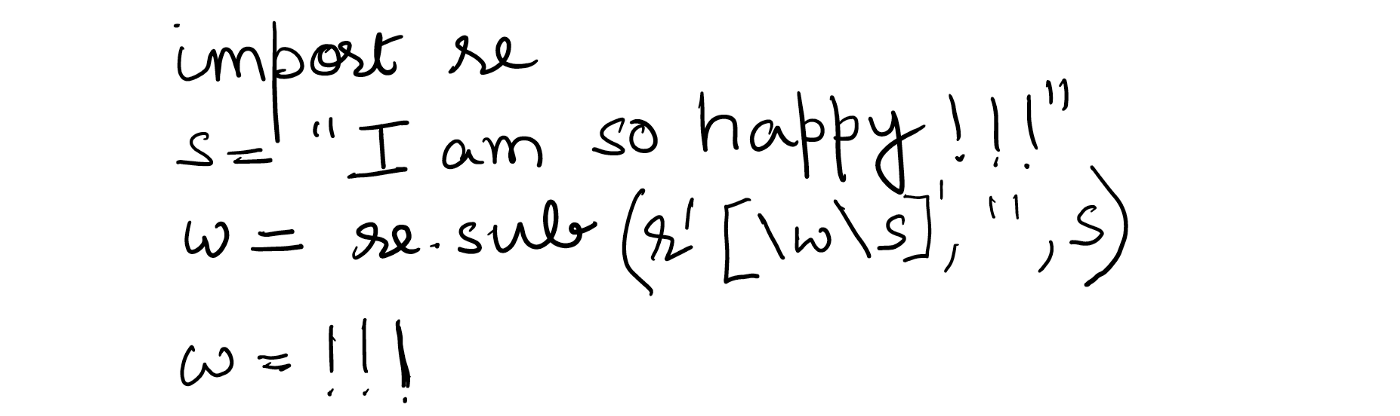However, with increasing complexity of language, the ability of rules-based systems like RE becomes difficult to generalize. For e.g. in complex spelling corrections, creating a rule to get the correct spelling could be very difficult and therefore, in such scenarios, we want to understand what the most likely correct spelling of the word could be. Let’s say there is a word: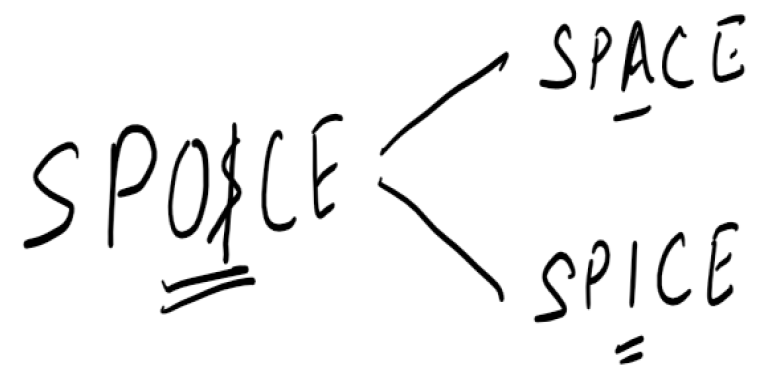Using RE, we can correct the spelling provided we know what the correct spelling is. If the correct spelling is not known, then we need to know the context in which this word has been used and then understand of the two words “Space” and “Spice”, which is more likely.

This is where probabilistic modeling come into the picture and works better than a rule-based system. Probabilistic modeling using statistical theory is more robust specifically with unfamiliar and erroneous input data. Let’s go through a few concepts in the context of Classification Tasks in NLP:

1.Text Classification e.g. Sentiment Classification

2.Sequence Modeling e.g. Part of Speech Tagging or Name entity recognition

Text Classification (e.g. Sentiment Classification)

In sentiment classification, the task requires categorizing any text e.g. movie/hotel reviews as positive or negative. This is a very widely used NLP application and let’s understand this through an example:

Below are some examples of comments from travelers about their travel experiences. The task here is to categorize a new comment as “Good” or “Bad” experience based on a given training data of past comments.

The labeled training data is given below:Here, X in the training data is all the comments from travelers and Y is the classes (2 classes: Good and Bad).

The task here is to develop a model on the training data, that in turn can predict which class any comment is likely to belong to.

So, for a new comment “Satisfactory visit”, the task is to predict the probability of its belonging to either class, i.e:A common technique used in this kind of task is Naïve Bayes, which, based on Bayes theorem, gives the joint probability of X and Y.

For those of you, who are not very familiar with probabilities — joint probability P(X,Y) gives the probability of both X and Y happening i.e. P (Y=good and X=satisfactory visit) or P (Y=bad and X=satisfactory visit). On the other hand, the conditional probability P(Y/X) is the probability of Y happening given that X has already happened. So, the task here is to find P(Y/X) as given in (A) above. Now, according to Bayes Theorem:

So, the task here is to find P(Y/X) as given in (A) above. Now, according to Bayes Theorem: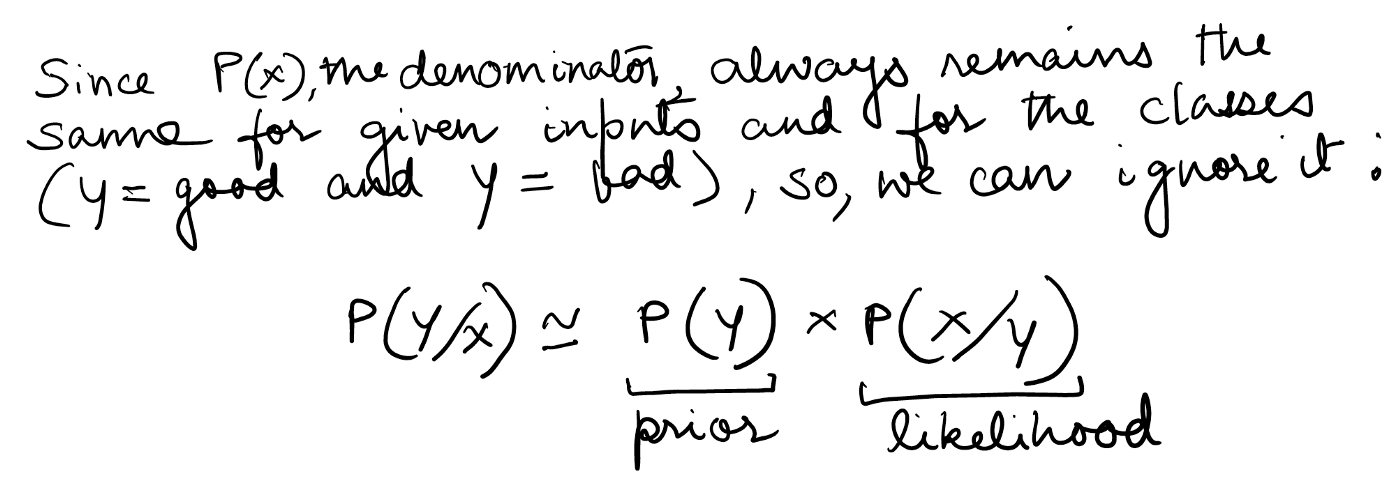So, the conditional probability of Y given X is the product of probability of Y (called prior) and the conditional probability of X given Y (called the likelihood). In the above example: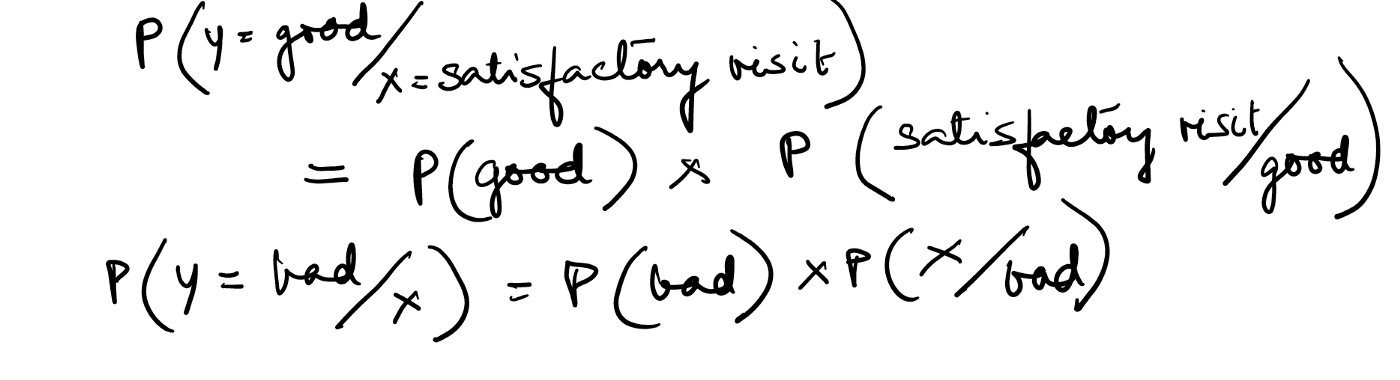Now, calculating the prior is easy (as we will see in the calculations below), it is the calculation of likelihood which can be difficult. Now, why could that be? X could be represented by features like x1,x2,x3…xn, and these features could be interdependent i.e. the probability of x1 belonging to a class {P(x1/class i)} could depend on the other features (x1, x2…xn) as well as their positions. Likelihood involves calculating the probability of the X (as represented by features x1, x2…xn) given a class: P (x1, x2, x3…xn/class i). If we do not assume independence of features of X to calculate this, we will have to consider all their interdependencies, which can be computationally very intensive and can become intractable. Hence Naïve Bayes makes the two assumptions:

• The position of the words in the sentence/text doesn’t matter.
• It assumes class conditional independence of x, i.e. the interdependence of the features of X is ignored and not considered while calculating the likelihood.

So, based on the above assumptions, we get: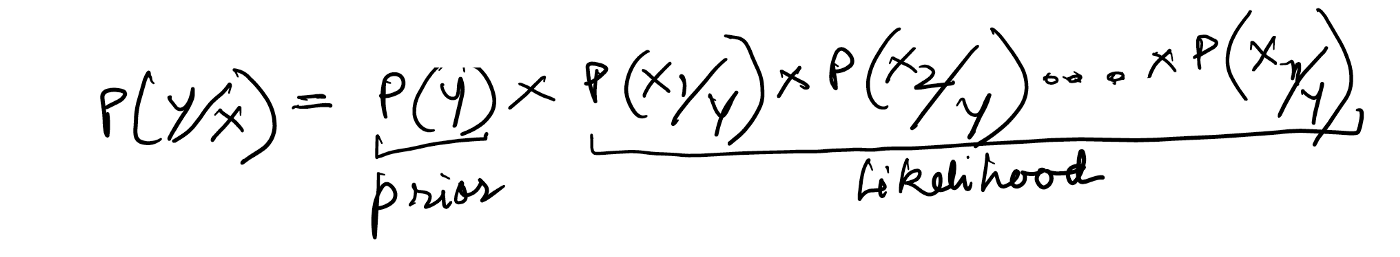Please note: According to probability theory, when we assume independence of features of X, the calculation of likelihood gets simplified just by taking the product of the conditional probability of features of X given Y.

Let’s now quickly come back to the example and calculate the probabilities: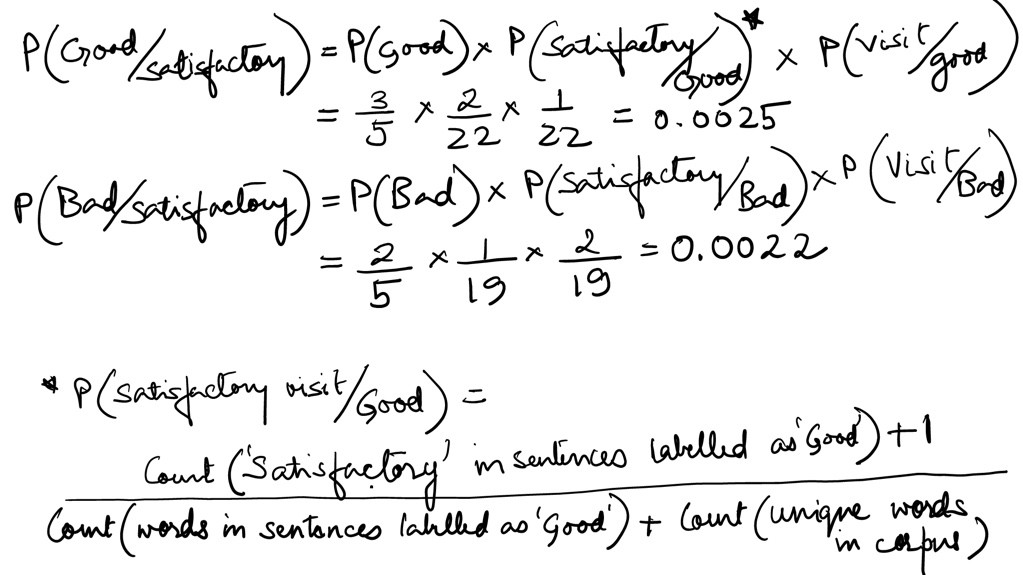Here, ‘1’ in the numerator is the smoothing factor

Although very small, the probability of “Satisfactory visit” belonging to “Good” is slightly higher than “Bad”.

A few key points to highlight above:

1.One of the biggest limitations of algorithms like Naïve Bayes is modeling the P(X/Y) — the likelihood. This is because the X could have highly dependent features and modeling them could become intractable. For e.g. sometimes in reviews, positive and negative sentences can appear together (like “NOT comfortable”). Since in Naïve Bayes, we assume independence of X and word position independence, we treat words individually leading to not consider the co-occurrence of these words.

2.Classifiers like Naïve Bayes let us use pre-defined distributions to generate the joint distribution of X and Y (for e.g. in many implementations of Naïve Bayes, P(x/y) is considered to follow a Gaussian distribution). So, if we understand the underlying distribution that defines the data well, we can generate the model and classify. However, if our assumption of the underlying data distribution doesn’t hold true, we might end up with a very bad classifier.

3.The Y classes (good and bad) as considered independent, which is a reasonable assumption for tasks like sentiment classification above (sentiment of a text is unlikely to be related to the sentiment of another text). However, in sequence modeling (which we will see later), interdependence of classes could be important to consider.

Naïve Bayes belongs to a class of classifiers called “Generative classifiers”, which basically models the joint distribution of P (X, Y). In other words, for the given input text, these classifiers give the probability of the class generating the input text. So, a generative classifier model can generate the entire data (X-inputs and Y-classes) by giving the joint distribution of Y (the class) and X (inputs) i.e. P (X, Y).

Generative classifiers have proved effective especially for small, sparse, unlabelled datasets. This is because generative classifiers allow us to use pre-defined distributions (e.g. Normal distribution) to generate the X and Y data, it does not really depend on the richness of the input data. This is also the reason why they are computationally more efficient.

However, generative classifiers also suffer from some serious limitations. Most notable is its requirement to model P(X/Y), which in turn requires handling the feature dependencies. Algorithms like Naïve Bayes assumes conditional independence of features, which reduces the effectiveness of the model. Another issue of overtly depending on modeling P(X/Y) is the situation of unseen data in the test set. For e.g. in the above case of travel experience sentiment classification, if the new sentence has words that are not in the training data, it can pose some serious challenges. Hence, in such cases, instead of modeling P(X/Y) for which we may not have any information, we would like to utilize other features like “words in text that are in capitals”, “number of punctuations” etc. that would help us classify.

This is where “Discriminative classifiers” come into the picture, where features of the input text are used to discriminate between classes. More formally, discriminative classifiers give the conditional distribution of Y given X i.e. P(Y/X). The biggest advantage of these classifiers is that they directly use the input features instead of modeling P(X/Y) to classify and since we are not trying to model the distribution of X, we are not concerned if they are correlated. So, when we have data with rich features (typically large, non-sparse data), and the objective is to improve the accuracy of the classification as a task, discriminative classifiers work much better.

One example of a discriminative classifier is Logistic regression, which would give P(Y/X) directly using features from the training data. In the example above, features that can be created like:

(a) x1=1, if there are capitalized words in the text, else 0.

(b) X2=1, if number of punctuations in the text >=1, else 0.

Now, the equation for logistic regression is:So, using these features and applying it to the equation for logistic regression, we get: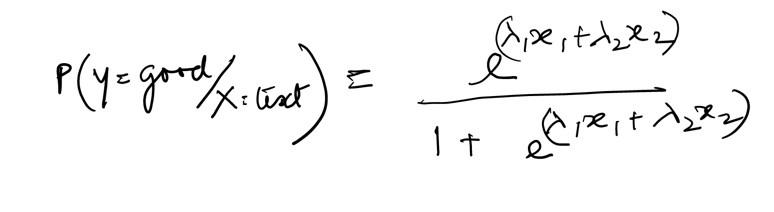As you can see, we are using the features directly to get the conditional distribution of Y given X and are not dependent on modeling P(X/Y).The parameters

The parameters of the model can be estimated using any optimization method like Gradient descent. Refer to the link to understand more on gradient descent: https://www.kdnuggets.com/2017/04/simple-understand-gradient-descent-algorithm.html

II. Sequence modeling (Part of Speech Tagging, Name Entity recognition)

In tasks like sentiment classification, one of the main assumptions is the independence of the output variables (Y). However, in many situations that may not hold true e.g. in sequence modeling. Let’s take a sentence:Now, the task here is to categorize each word of the sentence into various POS tags (e.g. Noun, Verb etc.). But as you can see that “eat” being a verb is very much dependent on the POS tag of “to”. Similarly, understanding the subject-predicate structure of sentences is important for POS tagging. Hence, in sequence classification, assuming the output classes as independent could be very difficult.

The classifiers that we have talked so far like Naïve Bayes, Logistic Regression and others like Hidden Markov models, Conditional Random Fields (which we will talk about later) can be represented in a framework called Probabilistic Graphical Models. They are specifically useful when modeling needs to consider distributions across many variables i.e. where output variable dependencies exists, as discussed above. The basic premise of these models is to represent these interdependent variables distributions through a product of local functions of smaller subsets of these variables. Let us understand this in detail using an example:

For a sentence like “The main city of UAE is Dubai”, the task is to classify each word into 4 classes: (a) y= ‘Location’ (b) y= ‘Person’ (c ) y= ‘Organization’ (d) y = ‘Others)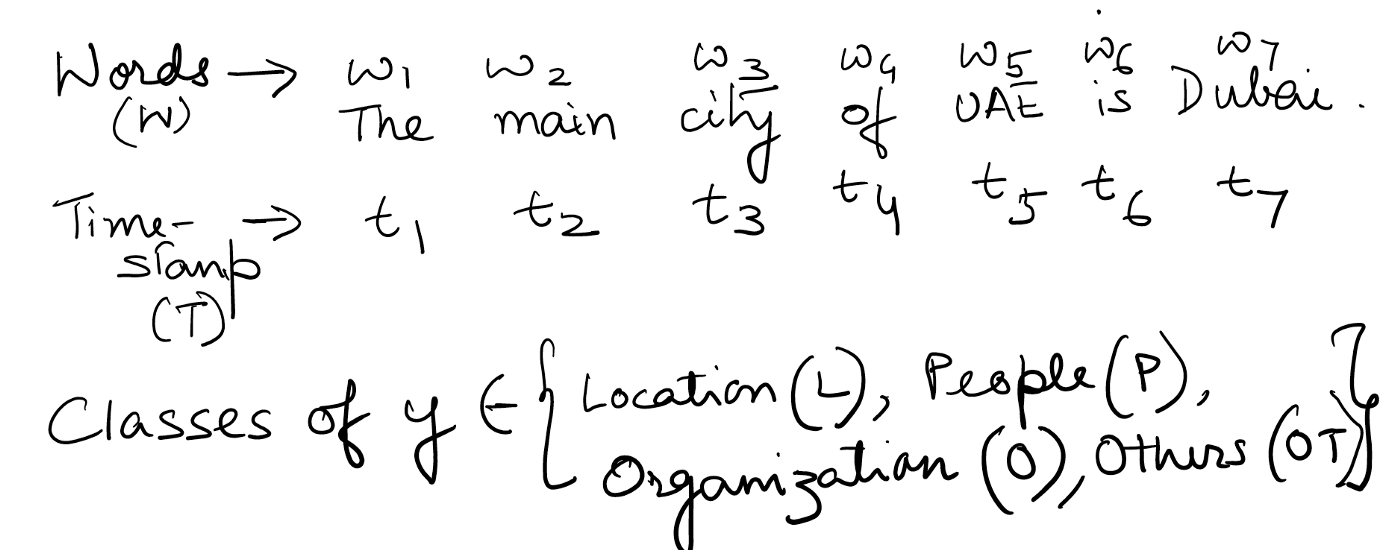Now, just like any other classifier, we create features of the input variables (W). In the context of graphical models, we construct local functions called compatibility functions based on feature functions that essentially describes relationships in smaller subsets of the variables to represent overall variable distribution. Examples of possible feature functions in the example:

•f1=1 if y=L and the sentence has words like {city, state}, else f1=0

•f2=1 if y=L and the y -1(class of previous word) is not a L, else f2=0

•f3=1 if y=P and if the previous word is a salutation, else f3=0

•f4=1 if y=O and y -1 NOT “L” or “P”, else f4=0

And so on…. As you can see above, the features here are not just text-based but also consider current and previous labels of words.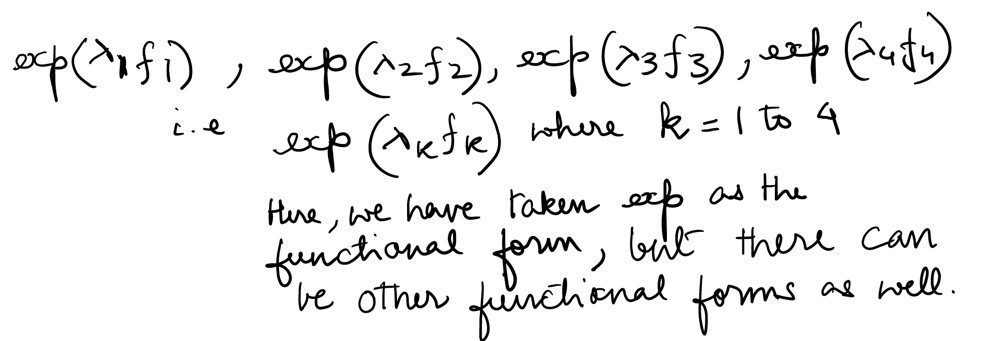Using the above, we can finally get the probability of every sequence possible, i.e.Finally, graphical models give the probability of any such sequence by the below equation: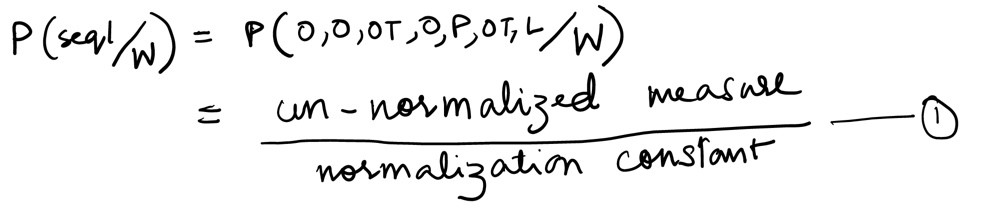Calculating the “un-normalized constant” or the numerator of (1):The “normalization constant” or the denominator of (1) is the summation of (a) or un-normalized measure across all possible sequences as given in (A):Finally, putting (a) and (b) together in (1), we get:Similarly, we calculate the probabilities of every possible sequence as given in (A) and tag the words in(W) as per the sequence whose probability is the highest.

Notes:

• To find the best values of parameters:we can use any optimization method like Gradient descent.

• The denominator of (B)i.e. normalization constant can become very large and intractable.Such methods can therefore be very computationally intensive.
• Refer to the Appendix to understand the full derivation of (B) using an example.

What we have outlined above in (B) is the equation of a sequence classifier called Conditional Random Fields (CRF). CRF is a discriminative sequence classifier, which has feature functions in the form of: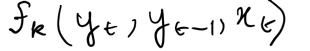and they have proved to be effective in many sequence classification tasks including deep learning.

The CRF that has been discussed above is a Linear-chain CRF, where the features are based on only the current and previous words labels: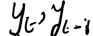However, general CRFs can also be created where features can be much richer by considering any word in the sequence. One variant of a general CRF is where the parameters at each timestamp (t) are different, which is unlike linear-chain CRFs, where the parameters mostly remain the same across t.

This wraps up the first section of the blog. In the second section, I am going to discuss recent advances in NLP algorithms including word embedding and deep learning.

References:

1. Speech and Language Processing: An Introduction to Natural Language Processing, Computational Linguistics, and Speech Recognition by Daniel Jurafsky and James H Martin
2. https://www.youtube.com/watch?v=rc3YDj5GiVM: Conditional Random Fields by Daphne Koller (Stanford University)
3. An Introduction to Conditional Random Fields by Charles Sutton and Andrew McCallum